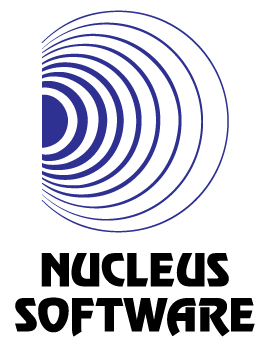Query multiples
Tag(s):

Binary search algorithm, Easy, Mathematics, Searching algorithm

Problem
Editorial
Analytics

An array $arr$ has indices numbered from 1 to N. You are given Q queries with two integers, $i X$ in each.

Write a program to find the number of multiples of X in the array $arr$ that falls between the $i^{th} index and the end of the array. Input format • First line: Two space-separated integers$N$and$Q$• Second line:$N$space-separated integers (denoting the array$arr$) • Next$Q$lines: Two space-separated integers,$i$and$X$Output format For each query, print the number of multiples of$X$in the array$arr$that falls between the$i^{th} index and the end of the array.

Constraints

$1 ≤ N ≤ 10^5$
$1 ≤ Q ≤ 10^5$
$1 ≤ X ≤ 20000$
$1 ≤ arr[i] ≤ 20000$

SAMPLE INPUT
3 1
9 6 2
2 2
SAMPLE OUTPUT
2
Explanation

There are two numbers, 6 and 2, after the index 2 divisible by 2.

Time Limit: 1.0 sec(s) for each input file.
Memory Limit: 64 MB
Source Limit: 1024 KB
Marking Scheme: Marks are awarded when all the testcases pass.
Allowed Languages: Bash, C, C++, C++14, Clojure, C#, D, Erlang, F#, Go, Groovy, Haskell, Java, Java 8, JavaScript(Rhino), JavaScript(Node.js), Julia, Kotlin, Lisp, Lisp (SBCL), Lua, Objective-C, OCaml, Octave, Pascal, Perl, PHP, Python, Python 3, R(RScript), Racket, Ruby, Rust, Scala, Swift, Swift-4.1, TypeScript, Visual Basic

CODE EDITOR

Initializing Code Editor...

This Problem was Asked inChallenge Name

Nucleus Software Coding Challenge

OTHER PROBLEMS OF THIS CHALLENGE
• Math > Number Theory
• Algorithms > Dynamic Programming